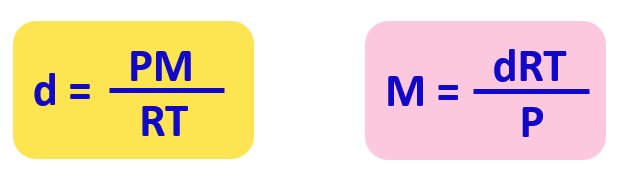## General Chemistry

Density and molar mass are calculated by modifying the ideal gas law equation. What we do is first replace the moles (n) in PV = nRT with mass over the molar mass:

${\rm{n}}\;{\rm{(moles)}}\,{\rm{ = }}\;\frac{{{\rm{m}}\;{\rm{(mass)}}}}{{{\rm{M}}\;{\rm{(molar}}\,{\rm{mass)}}}}$Next, we rearrange this formula to bring the V under the m which is the density of the gas (mass/volume = density):From this equation, we can obtain an expression for the density and molar mass of an ideal gas:## An example of calculating the density of an ideal gas:

What is the density of CO2 gas at 386 K and 17.0 atm.

${\rm{d}}\;{\rm{ = }}\;\frac{{{\rm{PM}}}}{{{\rm{RT}}}}$

${\rm{d}}\;{\rm{ = }}\;\frac{{{\rm{17}}{\rm{.0}}\;\cancel{{{\rm{atm}}}}\;{\rm{44}}{\rm{.0}}\;{\rm{g}}\;\cancel{{{\rm{mo}}{{\rm{l}}^{{\rm{ – 1}}}}}}}}{{{\rm{0}}{\rm{.08206}}\;{\rm{L}}\;\cancel{{{\rm{atm}}}}\;\cancel{{{{\rm{K}}^{{\rm{ – 1}}}}}}\;\cancel{{{\rm{mo}}{{\rm{l}}^{{\rm{ – 1}}}}}}{\rm{ 386}}\;\cancel{{\rm{K}}}}}\;{\rm{ = }}\;{\rm{23}}{\rm{.6}}\;{\rm{g/L}}$

## An example of calculating the molar mass of an ideal gas:

A scientist carries out an experiment to determine the molar mass of a 2.84-g sample of a colorless liquid which exerts 756 mmHg pressure when vaporized in a 260-mL flask at 142 oC. What is the molecular mass of this compound?

${\rm{PV}}\;{\rm{ = }}\;{\rm{nRT}}$

${\rm{PV}}\;{\rm{ = }}\;\frac{{\rm{m}}}{{\rm{M}}}{\rm{RT}}$

${\rm{M}}\;{\rm{ = }}\;\frac{{{\rm{mRT}}}}{{{\rm{PV}}}}$

${\rm{M}}\;{\rm{ = }}\;\frac{{{\rm{2}}{\rm{.84}}\;{\rm{g }}\;{\rm{0}}{\rm{.08206}}\;\cancel{{\rm{L}}}\;\cancel{{{\rm{atm}}}}\;\cancel{{{{\rm{K}}^{{\rm{ – 1}}}}}}\;{\rm{mo}}{{\rm{l}}^{{\rm{ – 1}}}}\;{\rm{415}}\;\cancel{{\rm{K}}}}}{{{\rm{0}}{\rm{.995}}\;\cancel{{{\rm{atm}}}}\;{\rm{0}}{\rm{.260}}\;\cancel{{\rm{L}}}}}\;{\rm{ = }}\;{\rm{374}}\;{\rm{g/mol}}$

Check Also

#### Practice

1.

Identify the unknown gas that weighs 17.75 grams in a 17.0 L cylinder held at 0.700 atm  pressure and 250°C.
a) NO2 b) CO2 c) H2 d) SO2 e) He

This content is available to registered users only. Click here to Register! By joining Chemistry Steps, you will gain instant access to the answers and solutions for all the Practice Problems and the powerful set of General Chemistry 1 and 2 Summary Study Guides.
Solution
This content is available to registered users only. Click here to Register! By joining Chemistry Steps, you will gain instant access to the answers and solutions for all the Practice Problems and the powerful set of General Chemistry 1 and 2 Summary Study Guides.
2.

What is the density of CO2 gas at 386 K and 17.0 atm.

This content is available to registered users only. Click here to Register! By joining Chemistry Steps, you will gain instant access to the answers and solutions for all the Practice Problems and the powerful set of General Chemistry 1 and 2 Summary Study Guides.
Solution
This content is available to registered users only. Click here to Register! By joining Chemistry Steps, you will gain instant access to the answers and solutions for all the Practice Problems and the powerful set of General Chemistry 1 and 2 Summary Study Guides.
3.

Determine the density of ammonia gas, NH3, at 36.0 oC and 695 mmHg. Report the density in grams per liter.